# “1 Day Like 1,000 Years”                                 and “1,000 Years Like 1 Day“

The theory of Relativity explains gravity based on the way space can ‘curve’, or, to put it more accurately, it associates the force of gravity with the changing geometry of space-time.

## Fractal Holographic Relativity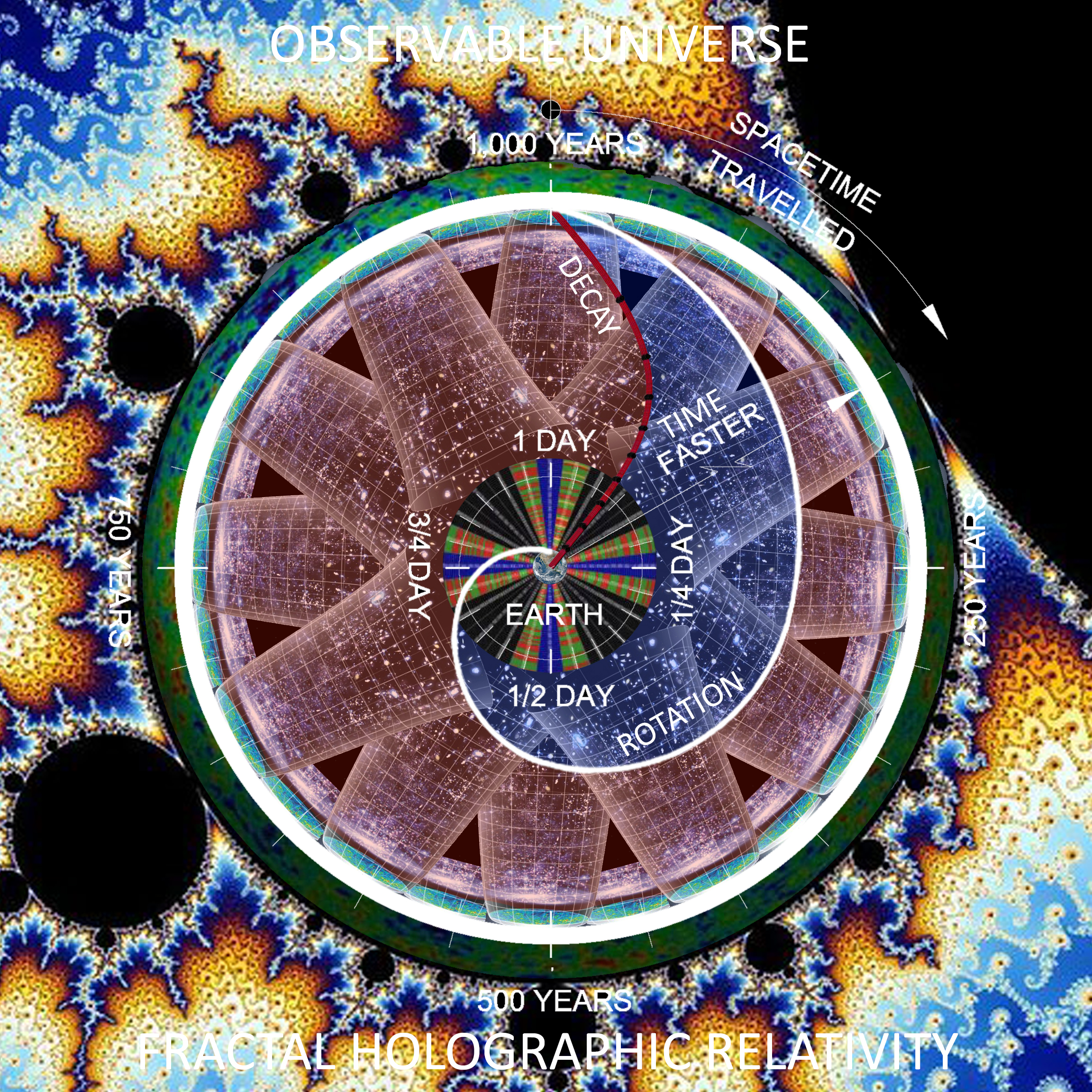### “Mathematics is the alphabet with which God has written the universe.” – Galileo Galilei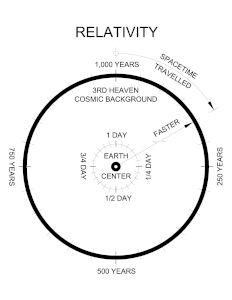2 Peter 3:8 But do not let this one fact escape your notice, beloved, that with the Lord one day is like a thousand years, and a thousand years like one day.

Psalm 90:4 For a thousand years in Your sight are like yesterday when it passes by, or as a watch in the night.# #FractalHolographicRelativity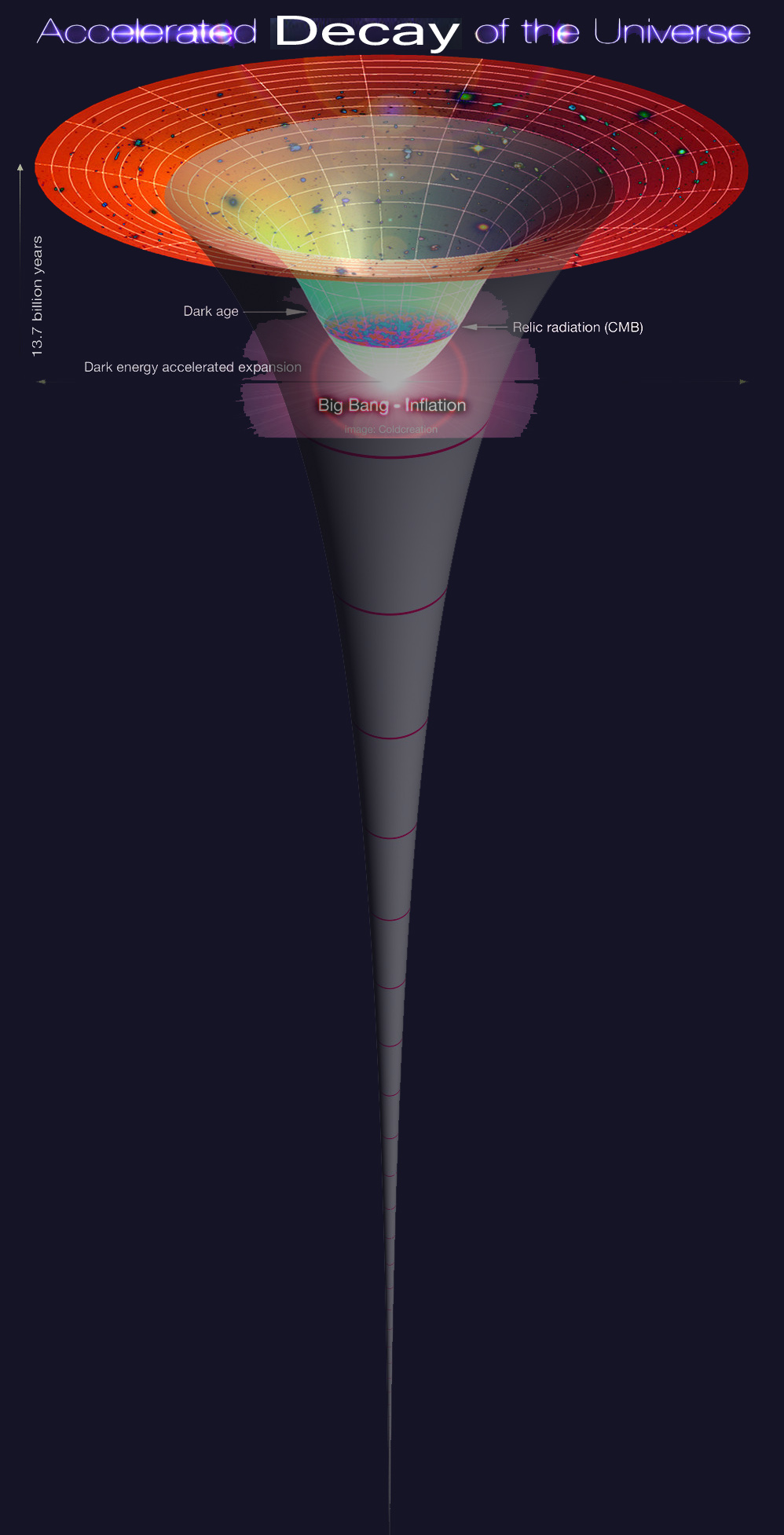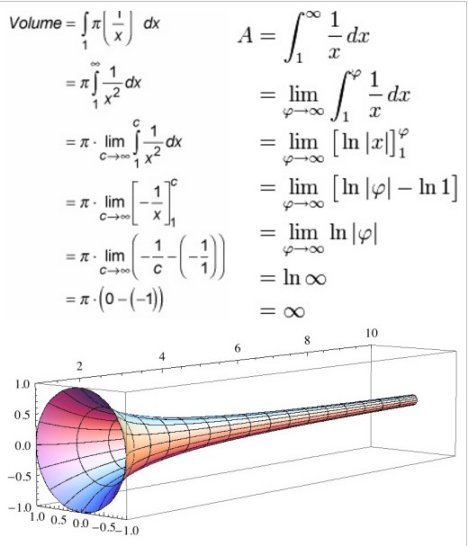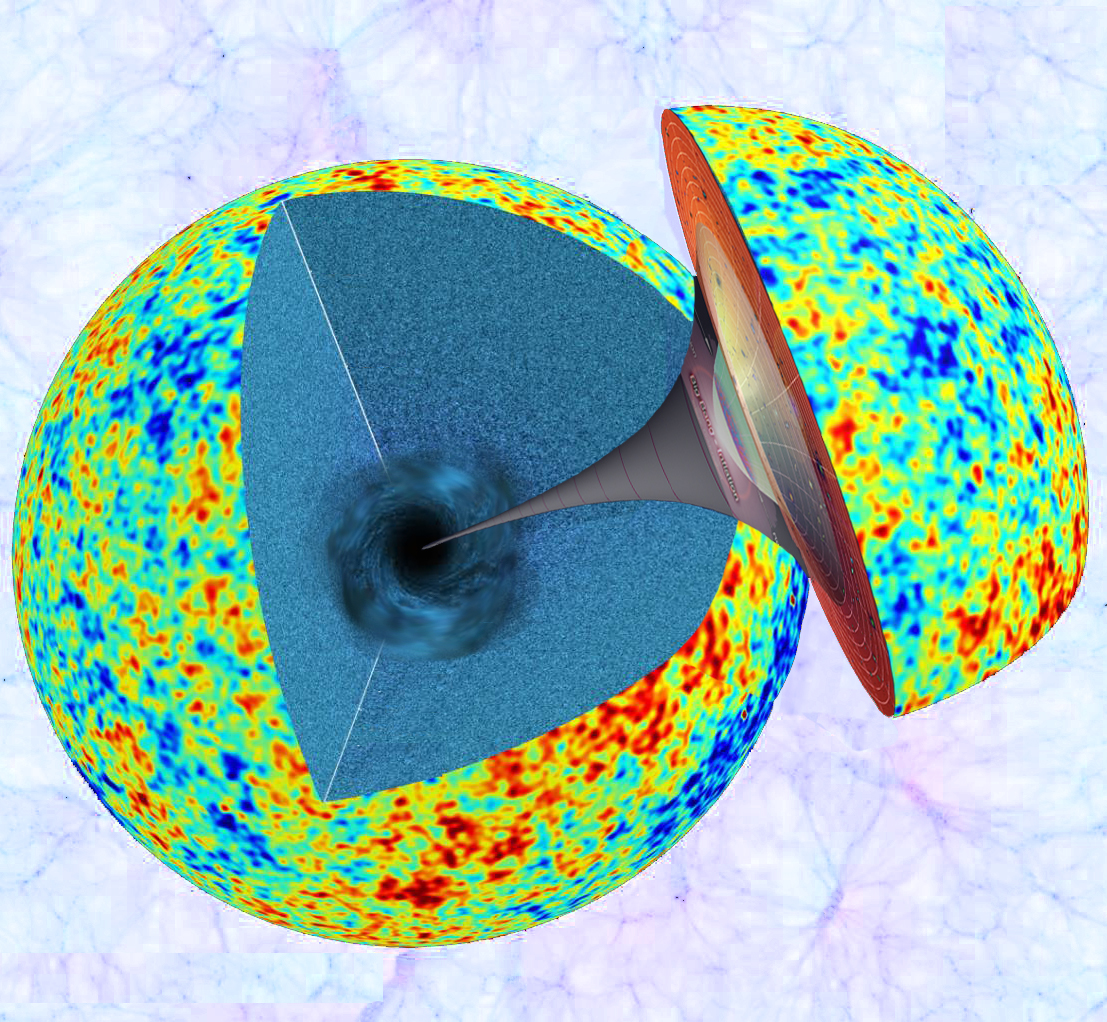Gabriel’s Horn

Gravity Probe-A

How many holes does it take to fill the Albert Hall . . ?

# SCIENTIFIC PROOF

WORK IN PROGRESS….

Known Fact:

It is a Scientifically Proven Fact, that Time Runs Faster, as measured on Atomic Clocks, the further the clock is Above the Earth.

Question:

How far Above the Earth, will the Time Dilation be “like” 1000 years Faster than 1 day on Earth?

Problem:

Solve the HYPOTHETICAL,  Relative Time Dilation at a distance Above the Earth, equivalent to the distance to the Cosmic Microwave Background.

Use a time dilation RATIO, extrapolated from existing Atomic Clock measurements, made from a known distance above the Earth , ie.  HAFELE-KEATING EXPERIMENT.

Given:

According to the HAFELE-KEATING EXPERIMENT, it is measured that an Atomic Clock run 273 +/-7 nanoseconds, (273e-9) seconds FASTER, at a distance of approximately 5.384 miles above, traveling around the Earth.

Definitions:

Nanosecond = 10−9 or 1/1,000,000,000 seconds

Speed of Light = 186,000 miles/second

Astronomical year (a single rotation around the sun) = 365.25 days.

Seconds in 1 year = 365.25 days = (365.25 days/year) × (24 hours/day) × (3,600 seconds/hour) = 31,557,600 seconds/year.

Age of Observable Universe = 13,800,000,000 years (presumed).

Time to Cosmic Background = 13,400,000,000 light years (measured?)

Distance to Cosmic Background = 13,400,000,000 light years x 186,000 miles/second x 31,557,600 seconds/year = 7.865e+22 miles.

Given ratios:

Altitude 0 miles : 1 day

Altitude 5.384 miles : +1/273,000,000,000 seconds (measured)

Altitude 7.865e+22 miles (CMB) : +1,000 years (t.b.d.)

Funny math:

1 x 7.865e+22 x 5.384 x (273e-9)^2  / 31,557,600 = 1,000

Einstein